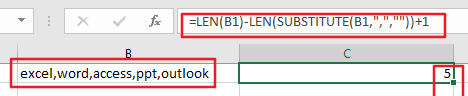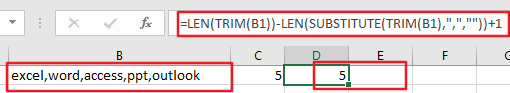# How to Count Comma Separated Value in One Cell in Excel

This post will guide you how to count values in a single cell separated by commas with a formula in Excel. How do I count comma separated values in one cell in Excel 2013/2016. Is it possible to have a formula that can count the amount of values separated by commas in a single cells in Excel.

## Count Comma Separated Value in One Cell

Assuming that you have a list of data which contain text string values, and each values is separated by comma character. For example, Cell B1 contains “excel,word,access”. I want to know how many values there are in Cell B1. The below method will show you how to count the number of values which are separated by comma character in a single cell.

Step1: you need to select a blank cell or the adjacent cell of Cell B1.

Step2: enter the following formula based on the LEN function and the SUBSTITUTE function in Cell C1, and press Enter key to apply this formula.

`=LEN(B1)-LEN(SUBSTITUTE(B1,",",""))+1`

Or you can use another similar formula to achieve the same result, like this:

`=LEN(TRIM(B1))-LEN(SUBSTITUTE(TRIM(B1),",",""))+1`

Step3: you would see that the total number of values separated by comma character is calculated in Cell C1.### Related Functions

• Excel Substitute function
The Excel SUBSTITUTE function replaces a new text string for an old text string in a text string.The syntax of the SUBSTITUTE function is as below:= SUBSTITUTE  (text, old_text, new_text,[instance_num])….
• Excel LEN function
The Excel LEN function returns the length of a text string (the number of characters in a text string).The syntax of the LEN function is as below:= LEN(text)…
• Excel TRIM function
The Excel TRIM function removes all spaces from text string except for single spaces between words.  You can use the TRIM function to remove extra spaces between words in a string.The syntax of the TRIM function is as below:= TRIM (text)….
Related Posts

Abbreviate Names Or Words in Excel

As an MS Excel user, you might have come across a task where you need to abbreviate different names or words, and there are also possibilities that you might have done this task manually by assuming that there isn't any ...

Extract Multiple Lines From A Cell

Suppose that you have listed some text in a single cell which is separated by the line break(you can do it by pressing ALT + ENTER after entering the text), and now you want to extract multiple lines of text ...

How to Remove All Extra Spaces and Keep Only One Between Words in Excel

Sometimes when copying something from other type files to excel, there might be two or more spaces display between words. Extra spaces between words are frequently to be seen in excel, and this behavior is very annoying. If we want ...

How to Remove Middle Name from Full Name in Excel

When we get a list of full names, we can remove the middle name for short in some cases. As the middle names are different among the full name list so we cannot replace them by space directly in excel. ...

How to Remove the First/Last Word from Text string in Cell

This post will guide you how to remove the first and the last word from a text string in cells in Excel. How do I use a formula to remove first and last word of a text string in Excel. ...

This post will guide you how to extract email address from a text string in Excel. How do I use a formula to extract email address in Excel. How to extract email address from text string with VBA Macro in ...

Remove Trailing Spaces from Cells in Excel

This post will guide you how to remove leading and trailing spaces in cells in Excel. How do I remove leading or trailing spaces in a range of cells with a formula in Excel. How to remove trailing spaces from ...

Convert Scientific Notation to Text or a Number in Excel

This post will guide you how to convert scientific notation to text or a number in Excel. How do I convert excel scientific to text with a formula in Excel. Convert Scientific Notation to Text or a Number in Excel ...

Reverse Concatenate Formula in Excel

This post will guide youw how to reverse the concatenate function in Excel. how do I split the text string into the muliptle cells with a formula in Excel. How to split text data of a cell to multiple cells ...

Remove Spaces from a String

This post will guide you how to remove extra spaces between words or text string from the specified range of cells in excel. How to delete all spaces from excel cells. For example, when you paste data from an external ...

Sidebar